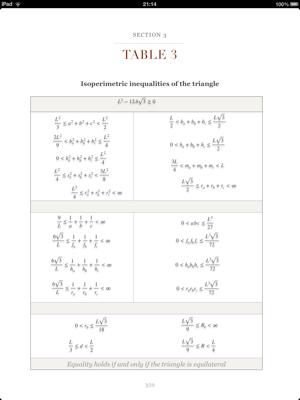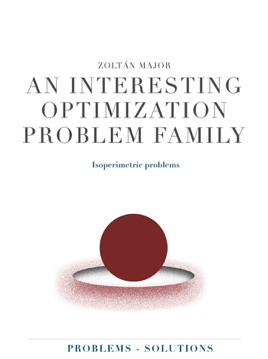• \$9.99

## Publisher Description

This book is primarily addressed to high school students and their teachers. It could be interesting also for university students studying mathematics and recommended to all those who have mathematical interest beyond the school curriculum.

The main goal of this book is to improve the problem-solving skills through the use of inequalities.

The book consists of 4 Chapters.

Chapter I - Theory

A brief overview of the classical isoperimetric problem, one of the earliest problems in geometry, that aroused the interest of lots of great mathematicians: Archimedes, Euler, Descartes, Lagrange, Minkowski, Weierstrass, Steiner and many others ...

Chapter II - Examples

The solved examples prepare the next chapters. You will find some typical wrong solutions in the notes which will help you avoid similar mistakes.

Chapter III - Problems

This chapter contains 237 specific isoperimetric and related problems in the 2- and 3-dimensional Euclidean space.

Chapter IV - Solutions

This chapter contains the solution on each of the 237 problems from the previous chapter.

The preferred method is the use of inequality where possible.

•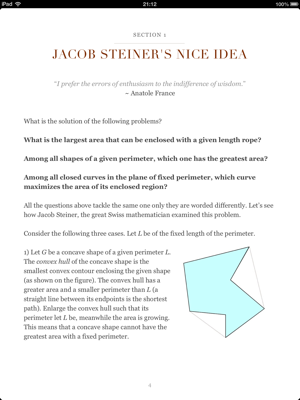•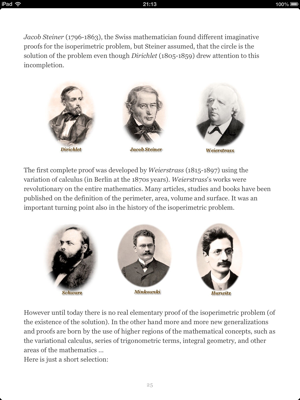•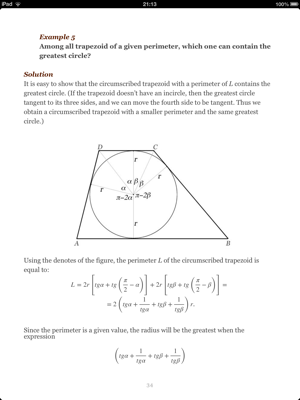•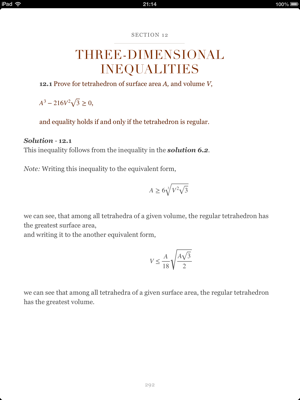•